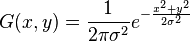## What is a Gaussian Blur?

Something I found fairly difficult to find online was a simple explanation on how to implement my own Gaussian Blur function. This article will explain how to implement one. The basic idea behind a Gaussian Blur is that each pixel becomes the average of the pixels around it, sort of. Instead of simply taking the average of all the pixels around it, you take a weighted average. The weighting of each pixel is greater if it is closer to the pixel you are currently blurring. The Gaussian Blur technique simply describes how to weigh each neighboring pixel. Imagine the pixel you are currently blurring is located at the peak of the hump in the image below and the pixels around it are receiving less weight as they get farther away. You can consider the image below to be considering up to 5 pixels away, this means the Gaussian blur has a ‘window’ of size 10, also known as a kernel size. This is where the Gaussian equation comes in, using it we can find out how much weight we want each pixel to receive and pixels receive less weight depending on its distance to the center pixel.Let’s explain what everything in this equation means: σ (lowercase sigma) – This is the blurring factor, the larger this number is, the smoother/blurrier the image becomes. e - This is simply euler’s number, a constant, 2.71828182846 x – This is the distance from the origin — The horizontal distance to the center pixel. y – This is the distance from the origin — The vertical distance to the center pixel. This means that x and y in this equation will be zero for the center pixel (the current pixel we want to blur), and x^2 + y^2 increases as we get farther away from the center, causing lower weights for pixels farther away.

## Calculating a Gaussian Matrix, also known as a Kernel

Let’s say we wanted to find out how we would weigh neighboring pixels if we wanted a ‘window’ or ‘kernel size’ of 3 for our Gaussian blur. Of course the center pixel (the pixel we are actually blurring) will receive the most weight. Lets choose a  σ of 1.5 for how blurry we want our image. Here’s what our weight window would look like:With each weighting evaluated it looks like this: (Notice that the weighting for the center pixel is greatest)If you’re pretty observant you’ll notice that this matrix doesn’t add up 1. For this to represent a weights, all the weights when summed together will have to add up to 1. We can multiply each number by 1/sum to ensure this is true. The sum of this matrix is 0.4787147. This means we need to multiply the matrix by 1/0.4787147 so that all elements end up adding up to 1. We finally get the following matrix which represents how we will weight each pixel during a blur.

## Applying this Gaussian Kernel Matrix to an image.

Lets say this is our image: (Each number can represent a pixel color from 0-255)To blur this image we need to ‘apply’ our kernel matrix to each pixel, i.e. we need to blur each pixel. Let’s say we want to blur pixel #25 (the pixel whose color is 25 in our image matrix). This means we get pixel 25 and replace 25 with the average of its neighbors. We weigh each of the neighbors (and 25 itself) with the kernel matrix we created earlier. So it would go as follows:Now that we have weighed each neighbor appropriately, we need to add up all the values we have and replace 25 with this new value! Loop this process with every single pixel and you will have a blurred image.

## Caveats

### Corner Pixels

When you need to apply this kernel matrix to a pixel in a corner, where you don’t have enough room to apply the matrix, a neat trick is to either ‘wrap around’ the image and use the pixels on the opposite side. This tends to work well if the image is intended to be tiled (typically not though) If you look at the image of the fish at the top of this article, you can tell that wrapping was used for the top row of the image since it is so dark. Another very simple solution is to just copy one of the nearest pixels into spots which are missing pixels so you can complete the process. The end result is definitely acceptable.

### Time Complexity

It turns out that the simple procedure described above can be improved greatly. The time complexity of the above algorithm is O(rows*cols*kernelwidth*kernelheight). Gaussian blur has a special property called separability, the blur can be applied to each kernel row first in 1 pass, then each kernel column in another and you can achieve the same result. This means that you do not need to traverse the entire kernel matrix for each pixel. This lowers the time complexity to O(rows*cols*kernelheight + rows*cols*kernelwidth). You can read more on the Separability property on the  Gaussian Blur wikipedia page. 稍候翻译，并给出小例子

© 著作权归作者所有### ifeixiang

2012/11/14
0
0

2008 年在一个 PS 讨论群里，有网友不解 Photoshop 的高斯模糊中的半径是什么含义，因此当时我写了这篇文章： 　　对Photoshop高斯模糊滤镜的算法总结； 　　在那篇文章中，主要讲解了高...

hoodlum1980
2015/05/25
0
0
(精选)EasyPR--中文开源车牌识别系统 开发详解（3）

03/28
0
0

tillusory
2018/01/31
0
0
SIFT四部曲之——高斯滤波

hit2015spring
2016/10/22
0
0

21分钟前
1
0

23分钟前
14
1

【技术沙龙002期】数据中台：宜信敏捷数据中台建设实践|宜信技术沙龙 将于5月23日晚8点线上直播，点击报名 一、关于Docker Docker 是一个开源的应用容器引擎，基于 Go 语言 并遵从Apache2.0...

25分钟前
9
0
Django框架配置

27分钟前
1
0
git代码迁移

28分钟前
1
0